## K-means in a “toy” dataset

Lets construct a more small but instructive example:

X = c(7, 3, 1, 5, 1, 7, 8, 5)
Y = c(1, 4, 5, 8, 3, 8, 2, 9)
rnames = c("x1", "x2", "x3", "x4", "x5", "x6", "x7", "x8")
kdata = data.frame(X, Y, row.names = rnames)

and plot the 2D dataset:

plot(kdata, pch = 15)
text(kdata, labels = row.names(kdata), pos = 2)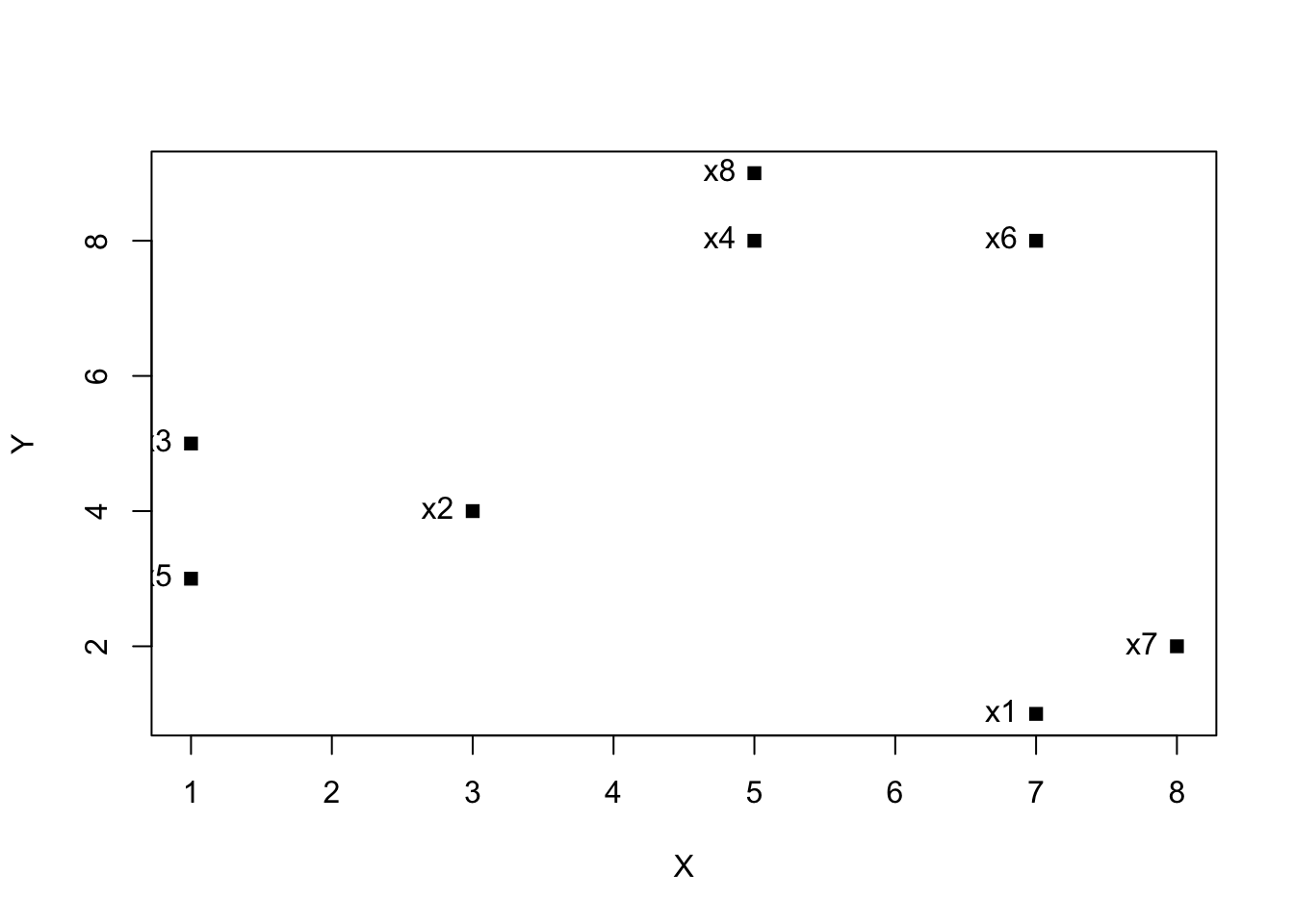### Create the clustering

# we take as initial centers the first 3 points and this implies also that k = 3
clust = kmeans(kdata, centers=kdata[1:3,])
clust$centers ## X Y ## 1 7.500000 1.500000 ## 2 5.666667 8.333333 ## 3 1.666667 4.000000 clust$cluster
## x1 x2 x3 x4 x5 x6 x7 x8
##  1  3  3  2  3  2  1  2

we can also easily retrieve metrics like cohesion and separation.

cohesion = clust$tot.withinss separation = clust$betweenss

and make a nice visualization

plot(kdata, col = clust$cluster, pch = 15) text(kdata, labels = row.names(kdata), pos = 2) points(clust$centers, col = 1:length(clust$centers), pch = "+", cex = 2)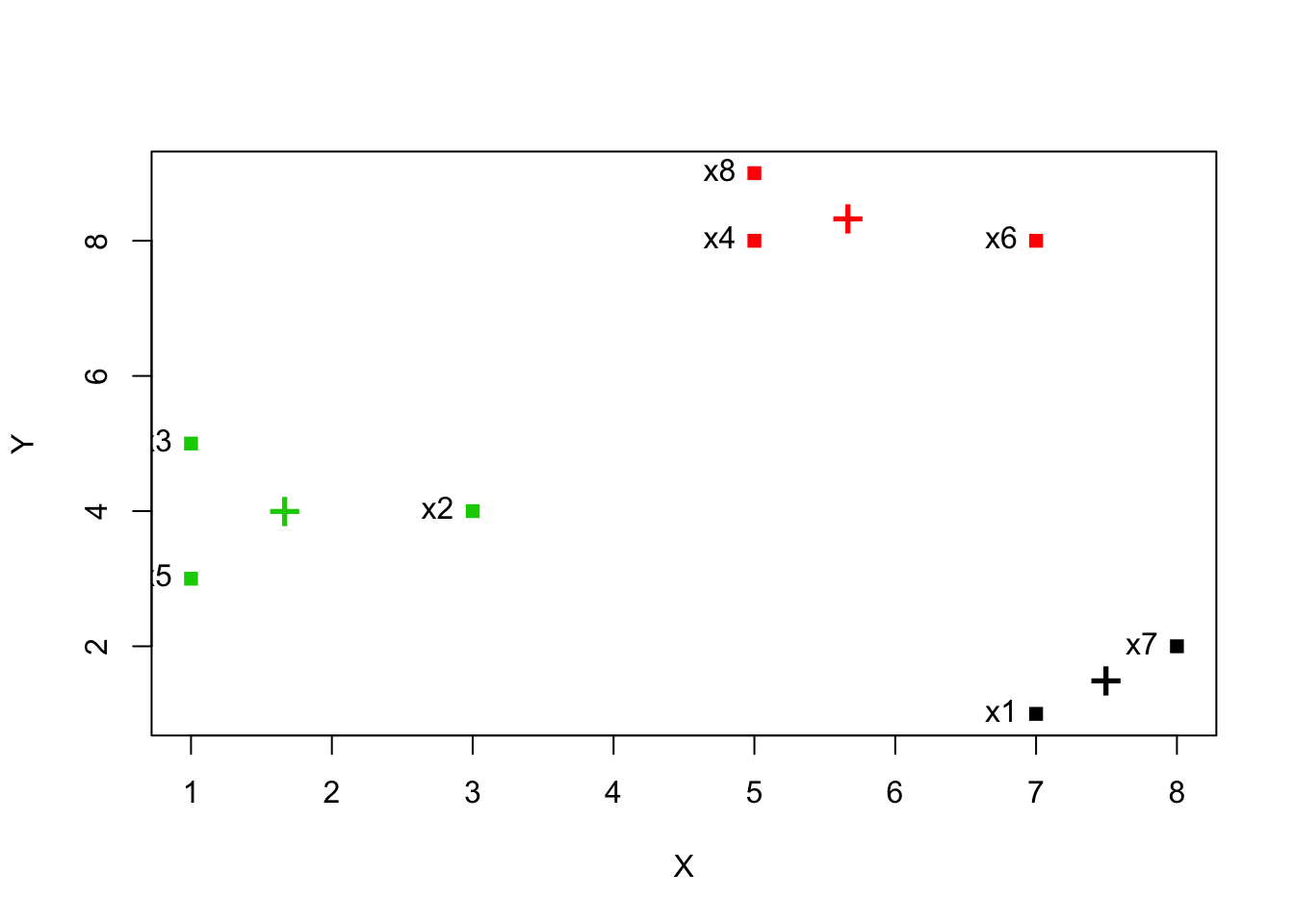## K-means for the iris dataset Lets apply the k-means clustering algorithm to the iris dataset. To begin with, we will exclude the Species column. data <- iris[,-5] clustering = kmeans(data, centers = 3) clustering ## K-means clustering with 3 clusters of sizes 62, 38, 50 ## ## Cluster means: ## Sepal.Length Sepal.Width Petal.Length Petal.Width ## 1 5.901613 2.748387 4.393548 1.433871 ## 2 6.850000 3.073684 5.742105 2.071053 ## 3 5.006000 3.428000 1.462000 0.246000 ## ## Clustering vector: ##  3 3 3 3 3 3 3 3 3 3 3 3 3 3 3 3 3 3 3 3 3 3 3 3 3 3 3 3 3 3 3 3 3 3 3 ##  3 3 3 3 3 3 3 3 3 3 3 3 3 3 3 1 1 2 1 1 1 1 1 1 1 1 1 1 1 1 1 1 1 1 1 ##  1 1 1 1 1 1 1 2 1 1 1 1 1 1 1 1 1 1 1 1 1 1 1 1 1 1 1 1 1 1 2 1 2 2 2 ##  2 1 2 2 2 2 2 2 1 1 2 2 2 2 1 2 1 2 1 2 2 1 1 2 2 2 2 2 1 2 2 2 2 1 2 ##  2 2 1 2 2 2 1 2 2 1 ## ## Within cluster sum of squares by cluster: ##  39.82097 23.87947 15.15100 ## (between_SS / total_SS = 88.4 %) ## ## Available components: ## ##  "cluster" "centers" "totss" "withinss" ##  "tot.withinss" "betweenss" "size" "iter" ##  "ifault" The clustering object contains a lot of informations and components: • The number of clusters (3) and their sizes • The centers of the 3 clusters • The clustering vector denoting which speciment (row) belongs to which cluster • The sum of squares of the distance between points and their centers for every cluster • total_SS is the sum of squared distances of each data point to the global sample mean • between_SS is the total_SS minus the the sum of the sum of square distances between points and their centers • The ratio will be close to 0 (0%) if there is no discernible pattern and closer to 1 (100%) if there is. • and other components like: # The centers clustering$centers
##   Sepal.Length Sepal.Width Petal.Length Petal.Width
## 1     5.901613    2.748387     4.393548    1.433871
## 2     6.850000    3.073684     5.742105    2.071053
## 3     5.006000    3.428000     1.462000    0.246000
# The clustering
clustering$cluster ##  3 3 3 3 3 3 3 3 3 3 3 3 3 3 3 3 3 3 3 3 3 3 3 3 3 3 3 3 3 3 3 3 3 3 3 ##  3 3 3 3 3 3 3 3 3 3 3 3 3 3 3 1 1 2 1 1 1 1 1 1 1 1 1 1 1 1 1 1 1 1 1 ##  1 1 1 1 1 1 1 2 1 1 1 1 1 1 1 1 1 1 1 1 1 1 1 1 1 1 1 1 1 1 2 1 2 2 2 ##  2 1 2 2 2 2 2 2 1 1 2 2 2 2 1 2 1 2 1 2 2 1 1 2 2 2 2 2 1 2 2 2 2 1 2 ##  2 2 1 2 2 2 1 2 2 1 # The total sum of squares (the sum of squared distances of each data point to the global sample mean) clustering$totss
##  681.3706
# The per cluster sum of squares
clustering$withinss ##  39.82097 23.87947 15.15100 # The sum of per cluster sum of squares clustering$tot.withinss
##  78.85144
# The total sum of squares minus the sum of per cluster sum of squares
clustering$betweenss ##  602.5192 # The sizes of the clusters clustering$size
##  62 38 50
# The number of iterations before conversion
clustering$iter ##  2 # Integer indicating possible algorithm problem clustering$ifault
##  0

### Comparing cluster to classes

For comparing the groupings provided by k-means with the actual classes we can use the table function:

table(iris$Species, clustering$cluster)
##
##               1  2  3
##   setosa      0  0 50
##   versicolor 48  2  0
##   virginica  14 36  0

With different initial centers in k-means one will get different values in the table above.

### Plotting

Lets also plot 2 dimensions of the iris dataset and visualize the clusters and their centers:

plot(iris[c("Sepal.Length", "Sepal.Width")], col = clustering$cluster) points(clustering$centers[,c("Sepal.Length", "Sepal.Width")], col = 1:3, pch = 8, cex=2)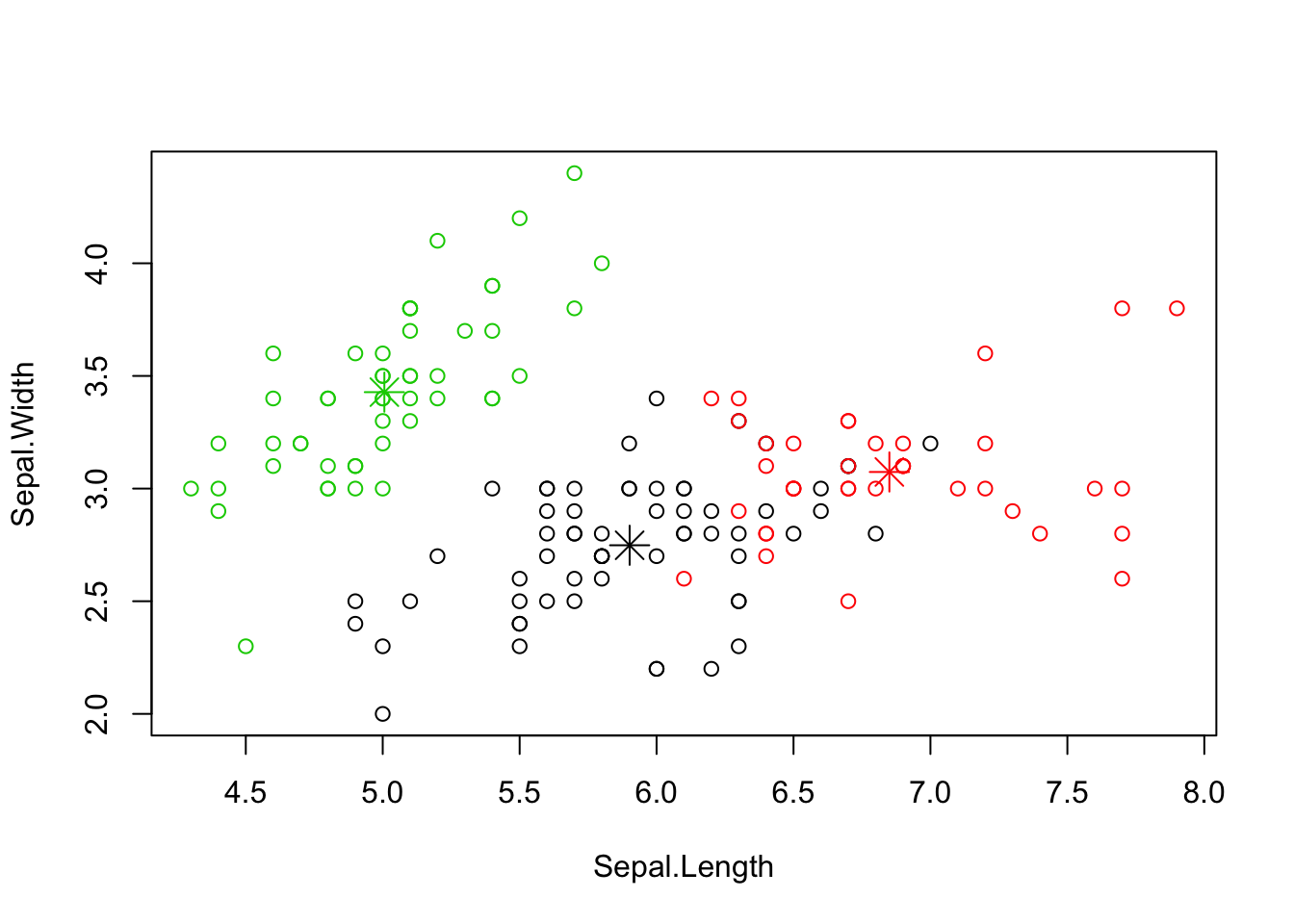### Selecting number of clusters

Returning to the iris dataset, a technique to find the number of clusters that describe the data better we can calculate the SSE (Sum of Squared Errors) for different number of clusters, say k = 1, 2, …, 10 etc. We can do that with the following commands:

# Calculate the totss (k = 1)
SSE <- (nrow(data) - 1) * sum(apply(data, 2, var))
for(i in 2:10) {
SSE[i] <- kmeans(data, centers = i)$tot.withinss } plot(1:10, SSE, type="b", xlab="Number of Clusters", ylab="SSE")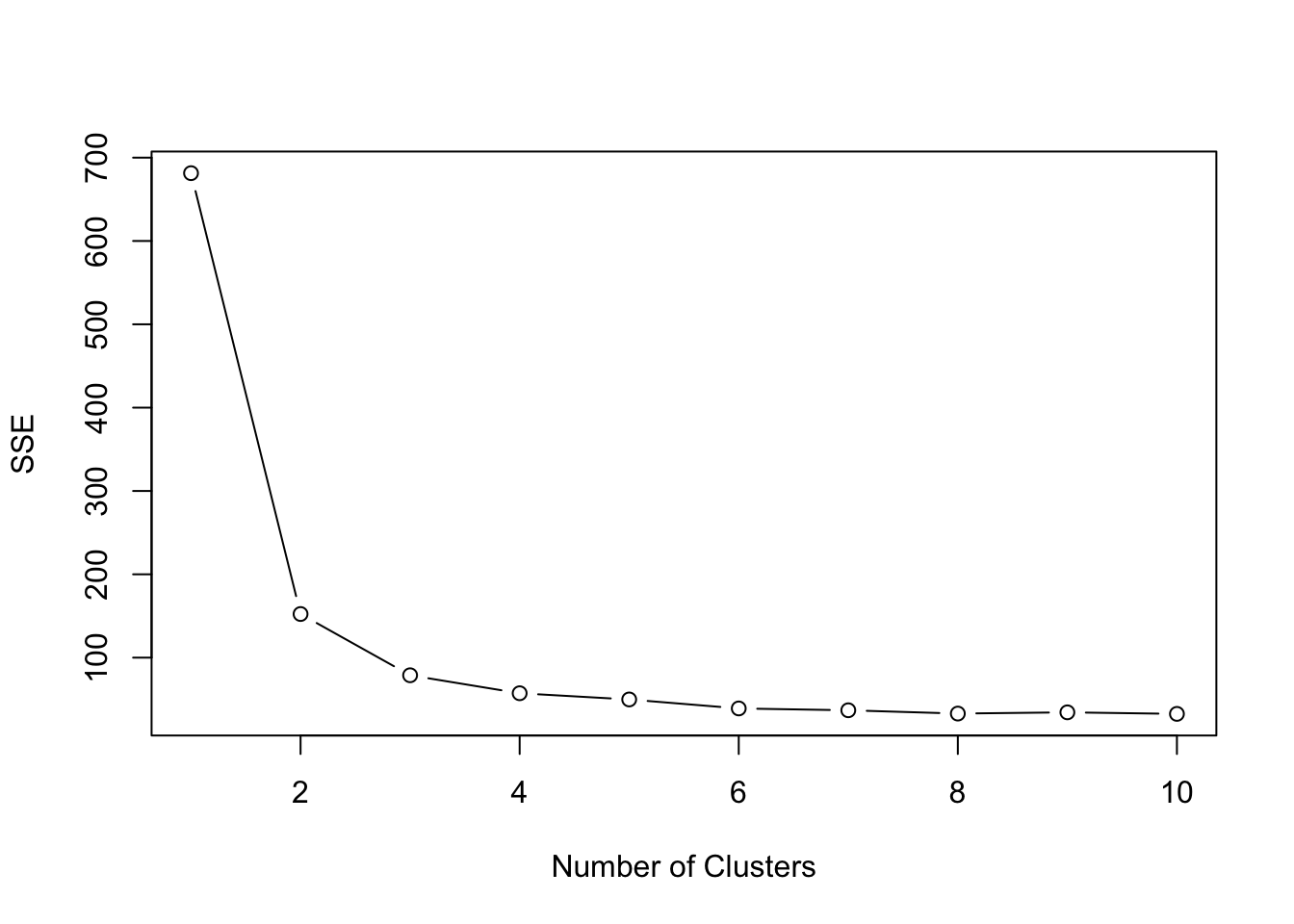We can see that k = 3 is a good pick and actually approximates the number of species available in the iris dataset. ### Silhouette coefficient To calculate the Silhouette coefficient we have to install and load the library cluster: # Assuming cluster library is installed with: install.packages('cluster') library(cluster) silhouette = silhouette(clustering$cluster, dist(data))
plot(silhouette)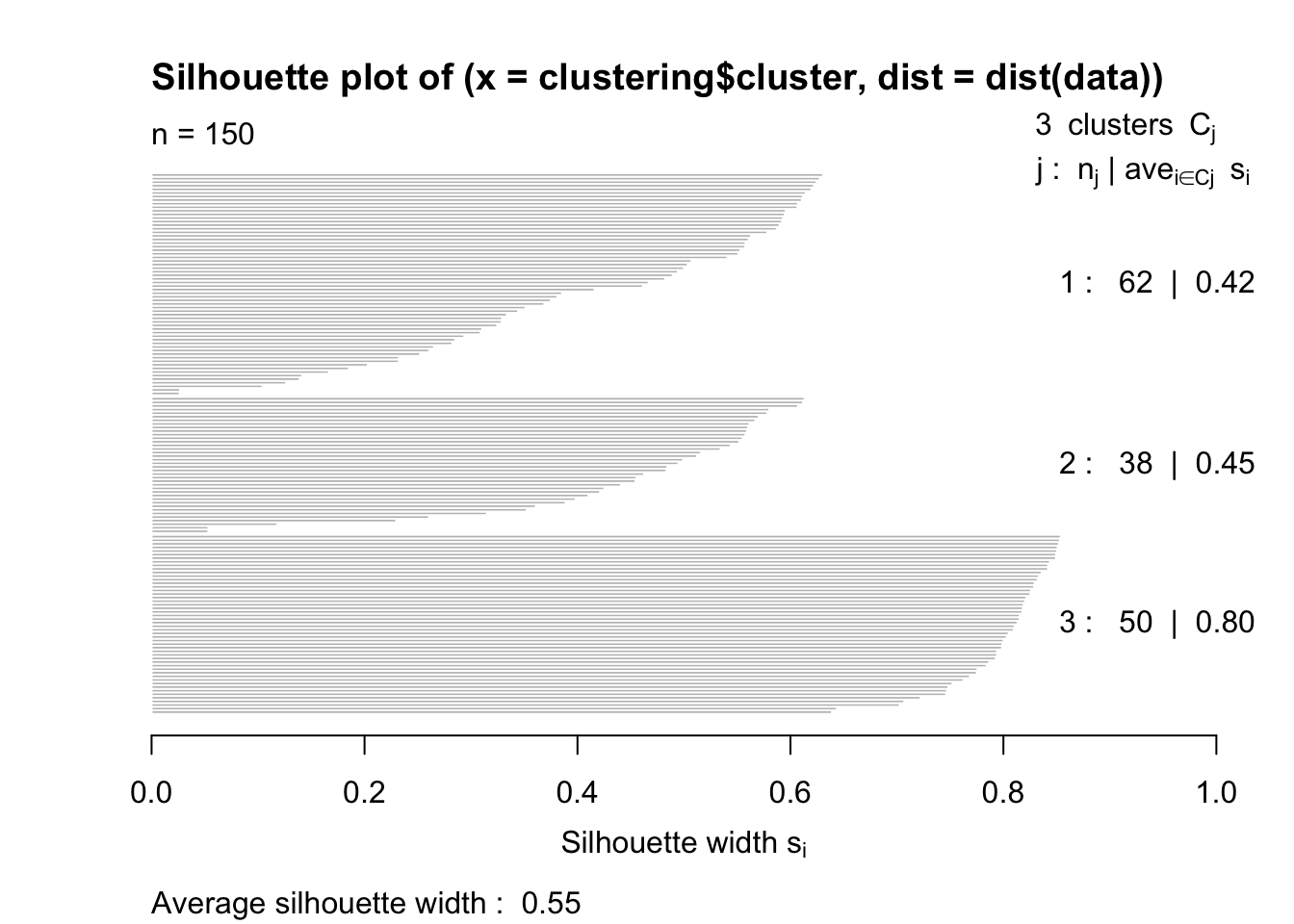The closer to 1 the better.